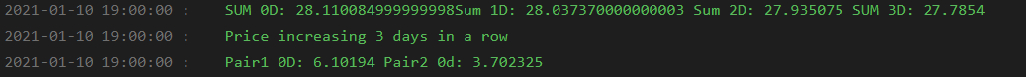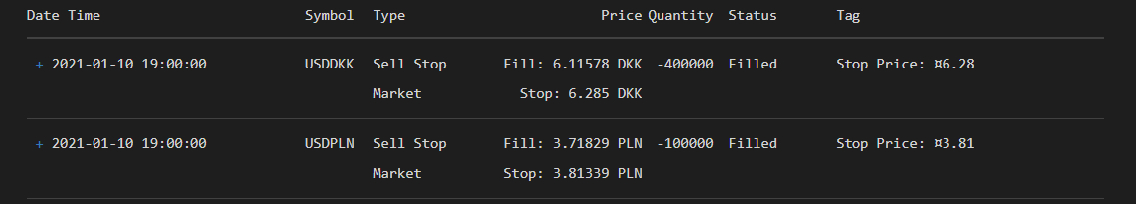Hello!

I implemented a simple mean reversion strategy. I sum prices of 2 FX pairs. Using rolling window I check if the sum was decling or increasing over the last few days. If the price goes down 3 days in a row I open a long position and hold for 2 days, and vice versa. Everything works well, as I receive correct signals to buy/sell pairs.

What concerns me however, is the difference between prices, which are printed from the RollingWindow function, and opening prices of my positions. Let me give you an example:Here as you can see, the price of the pair has been increasing 3 days in a row. “Sum 0D” so sum of the most recent FX prices is higher than “Sum 1D" so sum of yesterday's close, etc.

Then, I print the most recent prices of my FX pairs, using the rolling window function with a value of  at the end. The most recent price of the first pair is 6.10194 and price of the second pair is 3.70235. I receive these prices at 19:00:00 on 2021/01/10. After receiving this signal, the code then automatically sends sell orders, which are executed at the same time, at 19:00:00 on 2021-01-10However as you can see, the price at which my orders were executed, were significantly different to those obtained from rolling window function, which were supposed to be the most recent prices. Instead of opening a short USDDKK at 6.10194, the position was opened at 6.11578. Same issue with the second pair. Instead of being executed at 3.70235, it was shorted at 3.71829.

What could be the reason of such difference? Could it be caused by the difference in Bid and Ask? So that the rollingwindow shows the last price which could be for example mean of ASK and BID prices, however the trade is executed using BID prices, causing the divergence?

``````# region imports
from AlgorithmImports import *
# endregion

class CustomIndexStrategy(QCAlgorithm):

def Initialize(self):

self.Pair_1_Multiplier = 4
self.Pair_2_Multiplier = 1
self.Pair_1 = "USDDKK"
self.Pair_2 = "USDPLN"
self.holdingDays = 2

self.SetStartDate(2021, 1, 3)
self.SetEndDate(2022,6,24)
self.SetCash(1000000)
self.SetBrokerageModel(BrokerageName.OandaBrokerage)
self.SetBenchmark("SPY")
self.symbols = [self.Pair_1, self.Pair_2]
self.prevPrices = { symbol : RollingWindow[QuoteBar](7) for symbol in self.symbols }
self.ticketPair1 = None
self.ticketPair2 = None

def OnData(self,data):

for symbol in self.symbols:
if data.ContainsKey(symbol):

if not all([ window.IsReady for window in self.prevPrices.values() ]):
return

Pair1_window = self.prevPrices[self.Pair_1]
Pair1_6D = Pair1_window.Close
Pair1_5D = Pair1_window.Close
Pair1_4D = Pair1_window.Close
Pair1_3D = Pair1_window.Close
Pair1_2D = Pair1_window.Close
Pair1_1D = Pair1_window.Close
Pair1_0D = Pair1_window.Close

Pair2_window = self.prevPrices[self.Pair_2]
Pair2_6D = Pair2_window.Close
Pair2_5D = Pair2_window.Close
Pair2_4D = Pair2_window.Close
Pair2_3D = Pair2_window.Close
Pair2_2D = Pair2_window.Close
Pair2_1D = Pair2_window.Close
Pair2_0D = Pair2_window.Close

Sum_6D = (Pair1_6D * self.Pair_1_Multiplier) + (Pair2_6D * self.Pair_2_Multiplier)
Sum_5D = (Pair1_5D * self.Pair_1_Multiplier) + (Pair2_5D * self.Pair_2_Multiplier)
Sum_4D = (Pair1_4D * self.Pair_1_Multiplier) + (Pair2_4D * self.Pair_2_Multiplier)
Sum_3D = (Pair1_3D * self.Pair_1_Multiplier) + (Pair2_3D * self.Pair_2_Multiplier)
Sum_2D = (Pair1_2D * self.Pair_1_Multiplier) + (Pair2_2D * self.Pair_2_Multiplier)
Sum_1D = (Pair1_1D * self.Pair_1_Multiplier) + (Pair2_1D * self.Pair_2_Multiplier)
Sum_0D = (Pair1_0D * self.Pair_1_Multiplier) + (Pair2_0D * self.Pair_2_Multiplier)

if self.ticketPair1 is not None and self.UtcTime < self.ticketPair1.Time + timedelta(days=3):
return

if self.ticketPair1 is None and Sum_0D > Sum_1D > Sum_2D > Sum_3D:
self.Log(" SUM 0D: " + str(Sum_0D) + "Sum 1D: " + str(Sum_1D) + " Sum 2D: " + str(Sum_2D) + " SUM 3D: " + str(Sum_3D) + " SUM 4D: " + str(Sum_4D) + " SUM 5: " + str(Sum_5D) + " SUM 6D: " + str(Sum_6D) )
self.Log("Price increasing 3 days in a row")
self.Log("Pair1 0D: " + str(Pair1_0D) + "Pair2 0d: " + str(Pair2_0D) + "Pair1 1D: " + str(Pair1_1D) + "Pair2 1D: " + str(Pair2_1D))
self.ticketPair1 = self.StopMarketOrder(self.Pair_1, -100000 * self.Pair_1_Multiplier, 1.03 * self.Securities[self.Pair_1].Close)
self.ticketPair2 = self.StopMarketOrder(self.Pair_2, -100000 * self.Pair_2_Multiplier, 1.03 * self.Securities[self.Pair_2].Close)

if self.ticketPair1 is None and Sum_0D < Sum_1D < Sum_2D < Sum_3D:
self.Log(" SUM 0D: " + str(Sum_0D) + "Sum 1D: " + str(Sum_1D) + " Sum 2D: " + str(Sum_2D) + " SUM 3D: " + str(Sum_3D) + " SUM 4D: " + str(Sum_4D) + " SUM 5: " + str(Sum_5D) + " SUM 6D: " + str(Sum_6D) )
self.Log("Price decreasing 3 days in a row")
self.Log("Pair1 0D: " + str(Pair1_0D) + "Pair2 0d: " + str(Pair2_0D) + "Pair1 1D: " + str(Pair1_1D) + "Pair2 1D: " + str(Pair2_1D))
self.ticketPair1 = self.StopMarketOrder(self.Pair_1, 100000 * self.Pair_1_Multiplier, 0.97 * self.Securities[self.Pair_1].Close)
self.ticketPair2 = self.StopMarketOrder(self.Pair_2, 100000 * self.Pair_2_Multiplier, 0.97 * self.Securities[self.Pair_2].Close)

if self.ticketPair1 is not None and self.UtcTime >= self.ticketPair1.Time + timedelta(days = self.holdingDays):
self.Liquidate()
self.ticketPair1 = None
``````